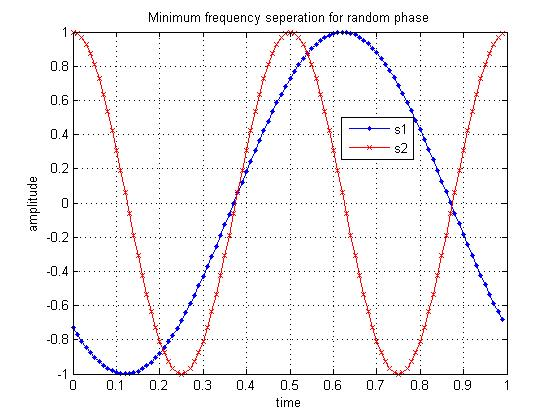# Minimum frequency spacing for having orthogonal sinusoidalsIn this post, the objective is to figure out the minimum separation between two sinusoidals having frequencies , of duration each to be orthogonal. Let the phase difference between the sinusoidals is where can take any value from to (Refer Example 4.3 [DIG-COMM-SKLAR]).

For the two sinuosidals to be orthogonal,

Integrating and applying the limits, the above equation simplifies to (thanks to the detailed simplification in Example 4.3 [DIG-COMM-SKLAR]) ,

.

Note:

and where is an integer.

Let as assume that is an integer. Then two terms in the above equation vanishes as

and .

The above equation simplifies to,

.

For an arbitrary value of from to

In a such a case, for the above equation to be zero, then the cosine term to be equal to 1 and the sine term need to be equal to 0 for making the above equation zero. To satisfy that requirement, need to have,

.

Ofcourse, the minimum value of is 1, then

.

For =

When = , then cosine term in the equation is already zero. To make the eqution 0, the sine term need to be equal to be zero. To satisfy that requirement, need to have,

.

Ofcourse, the minimum value of is 1, then

.

% Simple Matlab/Octave code

% Minimum frequency seperation between two sinusoidals

T = 1;
fs = 100;
t = 0:1/fs:T;
t = t(1:end-1);

% with random phase
f1 = 1;
f2 = 2;
phi = 2*pi*rand; % uniformly distributed from 0 tp 2pi
s1 = cos(2*pi*f1*t+phi);
s2 = cos(2*pi*f2*t);
sum_with_phi_random = sum(s1.*s2)

% with zero phase difference
f3 = 3/4;
f4 = 5/4;
s3 = cos(2*pi*f3*t);
s4 = cos(2*pi*f4*t);
sum_with_phi_zero = sum(s3.*s4)

close all
figure
plot(t,s1,’b.-‘)
hold on
plot(t,s2,’rx-‘)
legend(‘s1′,’s2’)
title(‘Minimum frequency seperation for random phase’)
grid on
xlabel(‘time’)
ylabel(‘amplitude’)

figure
plot(t,s3,’b.-‘)
hold on
plot(t,s4,’rx-‘)
legend(‘s3′,’s4’)
title(‘Minimum frequency seperation for zero phase’)
grid on
xlabel(‘time’)
ylabel(‘amplitude’)Figure: Two sinusoidals with frequency difference = 1/TFigure: Two sinusoidals with frequency difference = 1/2T

Summary

1. When the phase difference between two sinuosidals is not known, then the minimum frequency separation between them is for the sinusoidals to be orthogonal.

2. When the phase difference between two sinuosidals is zero, then the minimum frequency separation between them is for the sinusoidals to be orthogonal.

3. In the above Matlab code snippet, with seperation, the sum of the product of two sinusoidals is only nearly equal to zero (and not zero). Need to think more and revert.

References

[DIG-COMM-SKLAR] Digital Communications: Fundamentals and Applications (2nd Edition), Bernard SklarHope this helps,

Krishna

## 8 thoughts on “Minimum frequency spacing for having orthogonal sinusoidals”

1.fly4ever says:

3. In the above Matlab code snippet, with seperation, the sum of the product of two sinusoidals is only nearly equal to zero (and not zero). Need to think more and revert.

I think it is because that the Matlab sum function cannot present the real meaning of integrate, (the area of the curve of the product of two sinusoidals).
Hence
sum_with_phi_random = sum(s1.*s2)
should be add the factor 1/fs, as below:
sum_with_phi_random = sum(s1.*s2)*1/fs

2.ram says:

one more question it was mentioned that for what ever value of phi form 0 to 2pi the cos term should be one and the sin term is zero..

But it is not necessary…
check out for phi = 135degress and the cos term and sin term as 1 then even the equation goes to zero…..

3.ram says:

everything is ok. But, what about the condition (f1+f2)T is integer that is assumed in the derivation???

what about if (f1+f2)T is not integer???
???

1.Krishna Pillai says:

@ram: I did not do the detailed analysis, but I think (f1+f2)T being an integer is required for saying that two sinusoidals with zero phase difference to be orthogonal is f1-f2 = 1/2T

4.ram says:

everything is ok. But, what about the condition (f1+f2)T is integer that is assumed in the derivation???

what about if (f1+f2)T is not integer???

5.Krishna Pillai says:

@amr alaa: Thanks. You may subscribe to the RSS-over-email or RSS-feed 🙂

6.amr alaa says:

thanks
7.Rajesh.N says: